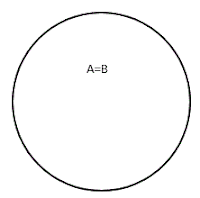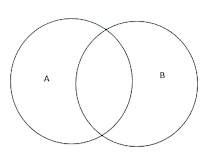IBPS Clerk 2021 Notification IBPS (Institute of Banking Personnel Selection) conducts a common recruitment process (CRP) every year to the r...

# Solving Syllogism using Venn diagram method

## Syllogism for bank exams

Solving syllogism is always confusing .No more confusion here onwards. Conventional methods are usually time consuming .It is okey if the problem is 2 statement syllogism. But it is not the case if the problem is 3,4 or 5 statement syllogism .Conventional method will eat your valuable time during exams. Please try to follow Venn diagram method explained below.
Before solving questions ,let us draw Venn diagram for possible statements.

1.Statement:”All A are B”
Possible Venn diagrams.fig1fig2

Let us see whether following conclusions follows ‘ the above statement  or not. A Conclusion follows if and only if conclusion is satisfied in all possible conditions of the statement. That means conclusion must be possible in all Venn  diagrams we have drawn .
>
You can see only conclusions 2 and 3  clearly  follows .But look at the conclusions 1 and 7,there is a possibility for these  conclusions to be true.If the conclusions 1 and 7 are modified as below
1=> All A being   B is a possibility(see fig.2). and
7=> Some B  not being  A is a possibility (see fig.1).
Then the answer will be’ YES’ and ‘YES’. Hope it is clear….Now let us go to next statement

2.Statement:”some A are B”
Possible Venn diagrams.fig.3

Let us see whether following conclusions follows the above statement or not.

You can see only conclusion 3 clearly follows. But in some cases there is possibility for conclusions 1,2,6 and 7 to be true. If we  modify 1,2,6 and 7 as follows.
1=> All A being B is a possibility.(see fig.4 and fig.6)
2=>All B being A is a possibility.(see fig.5 and fig.6)
6=>Some A not being B is a possibility(see fig.3 and fig.5)
7=>Some B not being A is a possibility(see fig.3 and fig.4)
Then these conclusions follows the statement.

3.Statement:"Some A are not B"
Possible Venn diagrams.

None of the conclusion follows directly. But there exist possibility for conclusion 2 to 7.If we modify them
2=>All B being A is a possibility? YES (fig.8)
3=>Some B  being A is a possibility? YES(fig.7 and fig.8)
4=>No A being B is a possibility ?YES(fig.9).
5=> No B being  A is a possibility ?YES (fig.9).
6=> Some A being  B is possibility?YES(fig.7 and fig.8).
7=> Some B  not being  A is a possibility.?YES(fig.7 and fig.9).
Hope you understood ….

4.Statement:"No A is B"
Possible Venn diagram

Conclusions 4,5 and 7 follows. In this case there is only one Venn diagram possible ,therefore there is no possibility cases.
Now let us do some simple problems.

Q1)Statement :Some Pens are Pencils.
All Pencils are Books.
Conclusions:1.All the Books are Pencil
2. Some Pens are Book.
3. All Pen being Book is a possibility.
or

Ans:1-NO,2-YES,3-YES.

Q2)Statement:Some Dogs are Cat.
Some Cats are Cows.
Conclusions:1.Some Dogs are Cows.
2. Some Cats are Dogs.
or

ANS:1-NO,2-YES

Q3):Statements:All cars are Chair.
No Chair is Mug.
Conclusions:1.No car is mug.
2.All Chair are cars.

ANS:1-YES,2-NO

[Tags:venn diagram syllogism examples,solving syllogism using venn diagram pdf,how to solve syllogism problems in reasoning using venn diagram,syllogism without venn diagram,syllogism possibility ,cases with venn diagram,syllogism without use of venn diagram,syllogism tricks for 4 statements,syllogism no case]

1.Statement

No fox is a cat
No cat is a dog
All monkeys are foxes

conclusion:
No monkey is fox
All dogs are definitely not cats
Some foxes are not dogs

which of following conclusions that does not follows?
using venn diagram

1.2.only 1 does not follows
2 follows because No cat is dog
Some foxes are not dogs also follows

3.4.5.thank you sir.. for best information

6.If there's a possibility, then the conclusion should be considered as follows or doesn't follow..?

7.If there's a possibility, then the conclusion should be considered as follows or doesn't follow..?

## Contact Form

Name

Email *

Message *

© 2015 bankAspire. WP Theme-junkie converted by Bloggertheme9